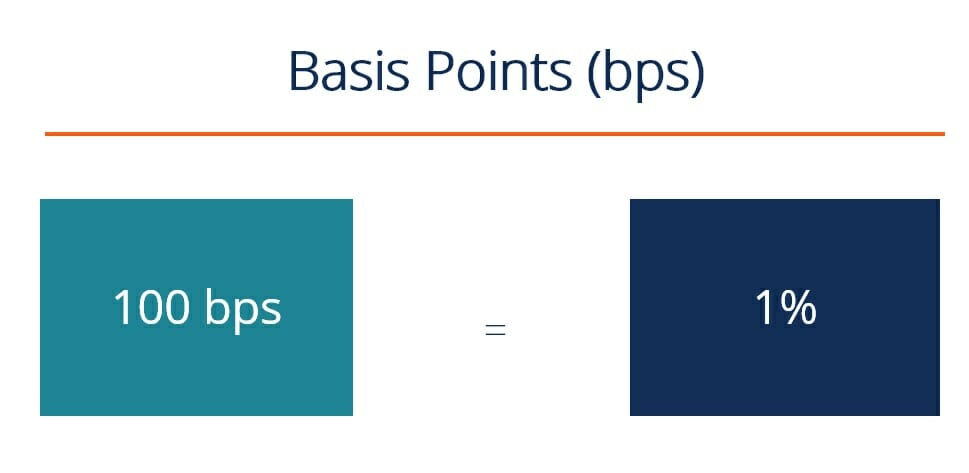# Basis Points (BPS)

One 1-hundredth of one percent

## What are Basis Points (BPS)?

In finance, Basis Points (BPS) are a unit of measurement equal to 1/100th of 1 percent.  BPS are used for measuring interest rates, the yield of a fixed-income security, and other percentages or rates used in finance.This metric is commonly used for loans and bonds to signify percentage changes or yield spreads in financial instruments, especially when the difference in material interest rates is less than one percent per year.

One basis point is equal to .01 percent or 1/100th of 1 percent. The succeeding points move up gradually to 100%, which equals 10000 basis points, as illustrated in the diagram below.

PercentageBasis Points
0.01%1
0.1%10
0.5%50
1%100
10%1000
100%10000

Examples:

• The difference between bond interest rates of 9.85 percent and 9.35 percent is 0.5 percent, equivalent to 50 basis points.
• The Federal Reserve boosts the interest rates at 100 BPS, signaling an increase from 10 percent to 11 percent.
• Due to the growth of iPhone sales, Apple Inc. reported high earnings, more than what was estimated; the stock increased 330 BPS, or 3.3 percent, in one day.

### Conversion between basis points and percentage

To convert the number of basis points to a percentage and, in turn, a percentage to basis points without using a conversion template or chart, review the following:

• Basis points to percentage – Divide the points by 100
• Percentage to basis points – Multiply the percentage by 100

### Why do investors and analysts use BPS?

The main reasons investor use BPS points are:

• To describe incremental interest rate changes for securities and interest rate reporting.
• To avoid ambiguity and confusion when discussing relative and absolute interest rates, especially when the rate difference is less than 1 percent, but the amount has material importance to discuss. For example, when discussing an interest rate that has increased from 11% to 12%, some may use the absolute method stating there is a 1% increase in the interest rate, while some may use the relative method stating a 9.09% increase in in the interest rate. Using basis points eliminates this confusion by stating that there is an increase in the interest rate by 100 basis points.

### What instruments do BPS apply to?

The usage of basis points is primarily applied to yields and interest rates, but they may also apply to the change in the value of an asset, such as the percentage changes of stock values. Other examples are:

• Treasury bonds
• Corporate bonds
• Interest rate derivatives
• Credit derivatives
• Equity securities such as common stock
• Debt securities such as mortgage loans
• Options, futures

• Simple interest
• Hurdle rates
• Line of credit
• Investment Banking
• Financial Modeling

### Financial Analyst Training

Get world-class financial training with CFI’s online certified financial analyst training program!

Gain the confidence you need to move up the ladder in a high powered corporate finance career path.

Learn financial modeling and valuation in Excel the easy way, with step-by-step training.